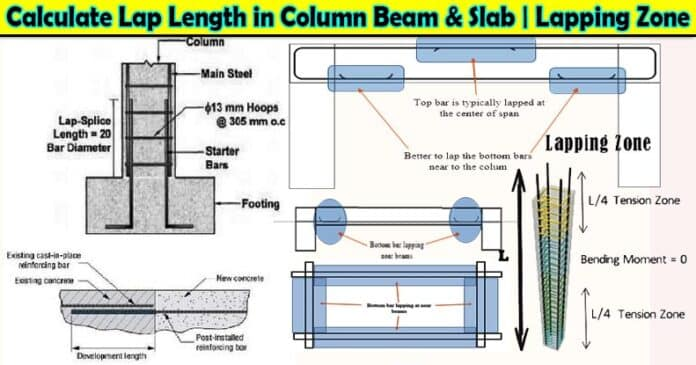# How to Calculate Lap Length of Rebar in Column Beam and Slab | Lapping Zone of Column Beam Slab and Footing:

In this Article today we will talk about the How to Calculate Lap Length in Column Beam & SlabLapping Zone of ColumnLapping Zone of BeamLapping Zone of Slab | Lap Length FormulaWhat is Lap Length | Common Lap Length as per IS 456 | Why we Provide Lap Length | Difference between Lap Length and Development Length of Rebar | Reinforcement Lap Length | Lap Length for Footing | Lap Length of Rebar

## What is Lap Length?

Lap Length is required, When bars are placed too short, they must be extended to the required length.

Lap length is the length provided to overlap two rebars (reinforcement) in order to safely transfer load from one bar to another bar and an alternative to this is to provide mechanical couplers. It is also called lap splices.

• When a bar diameter has to be changed along its length, Lap Length is also required. (as is sometimes done in columns).

The purpose of ‘Lap’ is to transfer the axial force from the terminating bar into the connecting bar with this same line of action (lateral) in the junction. This inevitably introduces stress concentrations at this surrounding concrete. These effects can be minimized by

• To avoid high flexural/shear stresses, keep lapping locations away from sections with these stresses. and

• Splicing the bars of a group at different locations (such as in a column).

When splicing becomes necessary in such situations, special precautions need to be taken., such as

• The lap length can be increased by using lap splices or welding.

• The stirrup design can include spirals or closely-spaced stirrups along the length of the stirrups.

### Overview:

When manufacturing reinforcement steel bars, engineers typically make them 12 meters long so they can be easily transported.

However, in the case of reinforced concrete structures, larger beams, columns and slabs often need to be made.

In such a case, the reinforcement bars have to be overlapped to get the required length.

Usually, such overlapping of reinforcement bars is done where the magnitude of the bending stress is least.

When the two reinforcement bars have an equal diameter; the lap length can be calculated using the following formula:

Lap Length = 50 x D

Where, D = diameter of the reinforcement bars

In the event that reinforcement bars do not have equal diameters, then the lap length is calculated using the value of the bar with a smaller diameter. Calculate Lap Length in Column Beam & Slab

#### a. Lap Length in Tension:

For the lap length in tension, the following formula can be used to calculate the lap length including the anchorage value of the hooks,

1. For flexural tension, Lap length = L x d or 30 x d (The greater value among the two values calculated is taken.)

2. For direct tension, Lap length = 2 x L x d or 30 x d (The greater value among the two values calculated is taken.)

Where,

L = development length

In such a case, the straight lapping length of the reinforcement bars must be greater than 200mm or 15 x d. Calculate Lap Length in Column Beam & Slab formula

#### b. Lap Length in Compression

For the lap length in compression, we can use the same value for the development length as well.

However, in no case, the lap length can be less than 24 x d.

## Why Do We Provide Lap Length of Rebar ???

Reinforcement steel is generally manufactured for 12 meters in length. During construction, when reinforcement is needed for a longer length than 12 meters, the bars are lapped to maintain continuity in the reinforced structures. The lap length varies in different RCC structures and generally lies between 30d (d=bar diameter) to 55d depending on the design and grade. Calculate Lap Length in Column Beam & Slab

### Importance of Providing Lap Length

The lap length is necessary for reinforced concrete structures to transfer tensile and compressive loads from one reinforcement bar to another by means of shear or skin friction.

If the lap length is not sufficient, the load transfer mechanism may be disrupted and lead to the failure of the entire structure. Lapping Zone of Column beam slab footing

Also, when the lap length provided is insufficient to reinforce concrete, the reinforcement bars may split, causing cracks in the concrete.

Thus, the lap length is necessary for reinforced concrete structures.

## What will happen if we don’t Provide Lap Length?

As we know that lapping should be provided between two rebars in order to safely transfer shear forces from one bar to the next bar, if we don’t provide lap length between two rebars the load is not safely transferred from one bar to the next bar, causing structure will be failed, if not failed it cause cracking, and permanent collapsing of a structure. For preventing any cracking, structure failure, and permanent collapsing, lapping should be provided in steel when we placed Steel in the RCC structure. Common Lap Length as per IS 456

## Lap Length As Per IS 456:

Generally, development length is 41d where d is the diameter of the bar. For direct tension, the lap length should be 2 Ld or 30d whichever is greater is considered. In this case, the straight length of the lapping bar shall not be less than 15d or 20cm. Common Lap Length as per IS 456

## Lap Length of Columns:

The codal provision for calculating the lap length of columns in a reinforced concrete structure is given in CL. 26.5.3 of IS 456:200.

According to this code, the diameter of the bars must not be less than 12mm.

In a rectangular column, the number of longitudinal bars must be four or greater and equal to or greater than six in a circular column.

The spacing of longitudinal bars should be less than 300 millimeters when measured along their periphery.

The lap length of columns can be calculated using the following formula,

Lap Length of Column = 45 x d

where; Common Lap Length as per IS 456

d = diameter of the bar Common Lap Length as per IS 456

#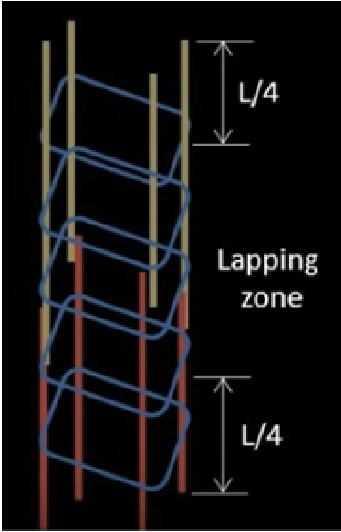• Lapping should be provided at the center of the column because the bending moment at mid-point is zero so try to lap at mid-point. Common Lap Length as per IS 456

• The lapping of bars should be provided alternately. Lap should not be given at the same point because buckling may occur.

• Lateral ties should be provided closely spaced in the Lapping zone.

• Due to maximum stress, we can’t lap at the column/beam/slab joint. Lapping should not be given at L/4 distance from the top and bottom of the slab. Why we Provide Lap Length

## Lap Length of Beams:

The codal provision for calculating the lap length of beams in reinforced concrete structure is given in CL. 26.5.2 of IS 456:200.

According to this code, side reinforcement bars must be provided in case the depth of the web of the beam is greater than 75 cm.

If a large percentage of the total area of a web is made up of reinforcement bars, then the reinforcement bars’ area must be greater than 0.1 percent of the total area of the web.

The reinforcement bars are to be equally distributed on both faces of the beam, then they must be spaced by no more than 300 mm or the web’s thickness, whichever is smaller.

In beams, the transverse reinforcements must be provided such that they lie around the exterior tension and compression bars.

In T-beams and I-beams, such reinforcement shall pass around longitudinal bars located close to the outer face of the flange. The lap length of beams can be calculated using the following formula, Why we Provide Lap Length

Lap Length of Beams = 60 x d

#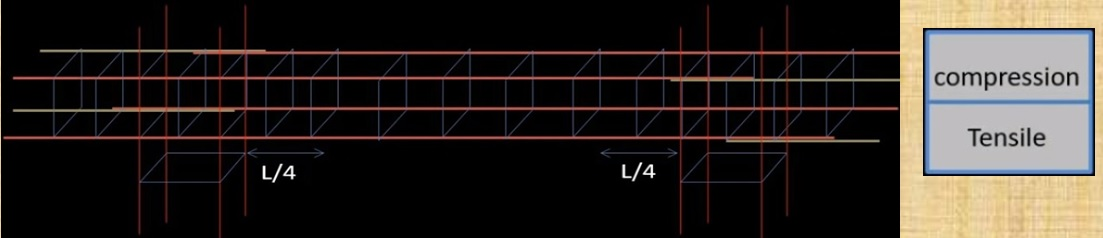• Lapping (24d) in top bars avoided L/3 distance from both ends. For the top bar, lapping should be at mid-span.

• Lapping (45d) in the bottom bars lap should be provided at column junction or L/4 distance from column face but should not be in mid-span of the beam.

• Stirrups should be closely spaced near the columns and lose/normal at mid-span.

• The lapping of bars should be alternately provided. Why we Provide Lap Length

## Lap Length of Slabs:

The codal provision for calculating the lap length of slabs in reinforced concrete structures is given in CL. 26.5.1 of IS 456:200.

According to this code, the diameter of the reinforcement bars must be lesser than one-eighth of the total slab thickness.

The lap length of slabs can be calculated using the following formula,

Lap Length of Slab = 60 x d Difference between Lap Length and Development Length

#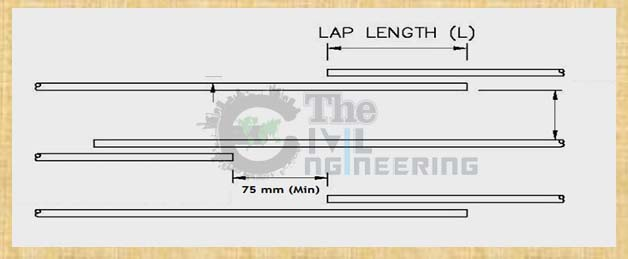(adsbygoogle = window.adsbygoogle || []).push({});

• RCC slab function is similar to beams if the slab is designed in one way. It is ideal to lap bars at the point of a least bending moment or at points of contra flexure.

• Practically laps are provided beyond L/5 to L/3 ( L being the effective span) from support for bars at bottom of the slab. The top bars are generally short and no laps are necessary. However, at no point, the lapped bars shall exceed one-third of the total bars.

• Bars will be considered staggered if end-to-end distance b/w laps is at least (lap length +75mm) Difference between Lap Length and Development Length

## Lap Length for Footing:

If you use dowels to reinforce concrete, the lap length will be 12D. If you are reinforcing with tension rods, then the lapping length will be 50D. This will depend on the diameter, coating and grade of steel used in your reinforcement project as well as the types of concrete you are working with. Reinforcement Lap Length

## What is Minimum Lap Length:

The minimum lap length for direct tension is equal to development length, it should be not less than 15d or 20cm. And for compression, the minimum lap length should be taken as not less than 24d. Where d is the diameter of rebar Reinforcement Lap Length

## Lapping Zone:

### Lapping Zone for Column:

This is the column. L is the length of the column. In the case of the column, the tension zone is located at an L/4 distance from both ends of the column. This zone experiences tension so here we should not provide lapping.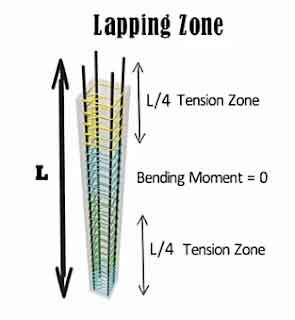Lapping Zone for Column

The bending moment at the middle portion of the column is zero which means the middle portion of the column is least stressed. Hence, lapping should be provided in the mid-section of the column so that transfer of stresses from bar to bar happens smoothly in this region. Lap Length for Footing

### Lapping Zone for Beam:

Whereas in the case of the beam as I already explained before the top portion of the beam experiences compression and the bottom portion experiences tension. So, the top reinforcement in the beam is left at the midspan. As the beam does not experience any negative moment at midspan so lapping is great in this region.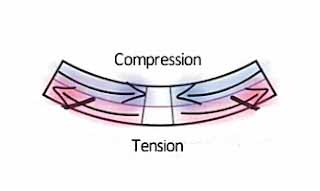Lapping Zone for Beam

In the case of bottom reinforcement, the lapping is provided near the ends of the beam or L/4  distance from column face but should not be in the midpoint of the beam, and one last point The lapping should not be provided at joints. Lap Length for Footing

### Lapping Zone for Slab: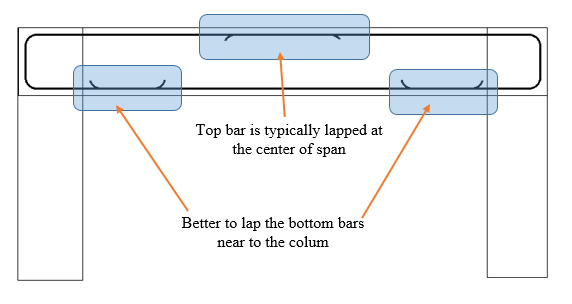Lapping Zone for Slab

The function of the RCC Slab is similar to the beam If it is designed in One Way Slab. The perfect position for lapping is at where the bending moment is least, the point of contra flexure. For bottom reinforcement, laps are provided L/5 to L/3 distance from the support. ( L = effective span) For top reinforcement, no lap is required since they are generally short. Although In no case, the lapped bars should not exceed one-third of the total bars.

In the two-way slab, the same practice as mentioned above should be followed for both directions.

If an end-to-end distance between the lap is the least, then the lap should be provided in a staggered manner. ( lap length +75 mm)

Generally, the lap length provided for the slab 60d, column 45d, and the beam is 60d for the M20 grade of concrete. Lap Length for Footing

## Difference Between Lap Length and Development Length:

 S.N. Lap Length Development Length 1 It refers to the overlapping length of two reinforcement bars for achieving the desired design length. It refers to the length that is necessary for transferring the stress imposed on the concrete member. 2 Lapping is done in reinforced concrete structures, to achieve the required design length of the reinforcement bars. Development length is necessary to ensure that the necessary bond strength between the concrete and the embedded reinforcement bar is achieved.

## FAQs:

### Q. Determine the lap length for two bars with a diameter of 40mm.

Solution,

When the two bars have the same diameter, the lap length can be calculated as,

Lap Length = 50 x d = 50 x 40 = 2000 mm = 2 m

### Q. Determine the lap length for two bars in which the diameter of one bar is 25mm and another of diameter 40mm.

Solution,

When two bars have a different diameter, the smaller diameter must be used i.e.

Lap Length = 50 x d = 50 x 25 = 1250 mm = 12.5 m

### Q. Determine the lap length for beam, slab (Take the diameter of bar = 12 mm) and the lap length for column (Take the diameter of bar = 24 mm).

Solution,

i. Lap Length of beam = 60 x d = 60 x 12 mm = 720 mm

ii. Lap Length of slab = 60 x d = 60 x 12 mm =720 mm

iii. Lap Length of column = 45 x d = 45 x 24 mm = 1080 mm

### Q. Determine the lap length for the following:

i. Nominal Mix of 1:2:4, if the diameter of the bar is 20 mm.

Lap Length for 1:2:4 concrete mix = 40 x D = 40 x 20 mm = 800 mm

ii. Nominal Mix of 1:1.5:3 for the column, if the diameter of the bar is 20 mm.

Lap Length for 1:1.5:3 concrete mix for column = 45 x D = 45 x 20 mm= 900 mm

iii. Nominal Mix of 1:1.5:3 for beam, if the diameter of the bar is 20 mm.

Lap Length for 1:1.5:3 concrete mix for beam = 60 x D = 60 x 20 mm = 1200 mm

iv. Nominal Mix of 1:1.5:3 for the slab, if the diameter of the bar is 20 mm.

Lap Length for 1:1.5:3 concrete mix for slab = 60 x D = 60 x 20 mm = 1200 mm

## Conclusion:

Full article on Lap Length in Column Beam & Slab | Lapping Zone of Column | Lapping Zone of Beam | Lapping Zone of Slab | Lap Length Formula | What is Lap Length | Common Lap Length as per IS 456 | Why we Provide Lap Length | Difference between Lap Length and Development Length | Reinforcement Lap Length | Lap Length for Footing. Thank you for the full reading of this article in “The Civil Engineering” platform in English. If you find this post helpful, then help others by sharing it on social media. If any formula of BBS is missing from this article please tell me in comments.

1.Musembi Malinda
2.ELMER ANDRIAMIZAKA